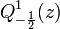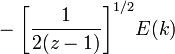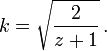User:Tohline/Math/EQ Q1minusHalf01$~Q^1_{-\frac{1}{2}}(z)$$~=$$~ - \biggl[\frac{1}{2(z-1)} \biggr]^{1 / 2} E(k)$ where:$~k = \sqrt{ \frac{2}{z+1}} \, .$ (see our associated derivation)